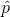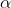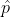##### Question

In: Statistics and Probability

# Assume that a sample is used to estimate a population proportion p. Find the margin of...

Assume that a sample is used to estimate a population proportion p. Find the margin of error E that corresponds to the given statistics and confidence level. Round the margin of error to four decimal places. 95% confidence; the sample size is 9900, of which 30% are successes.

## Solutions

##### Expert Solution

Solution :

Given that,

n = 9900

Point estimate = sample proportion == 0.30

1 -= 1- 0.30 =0.70

At 95% confidence level the z is ,= 1 - 95% = 1 - 0.95 = 0.05/ 2 = 0.05 / 2 = 0.025

Z/2 = Z0.025 = 1.96   ( Using z table )

Margin of error = E = Z/2   * (((* (1 -)) / n)

= 1.96 (((0.30*0.70) / 9900)

=0.009027

Margin of error = E =0.0090

## Related Solutions

##### Assume that a sample is used to estimate a population proportion p. Find the margin of...
Assume that a sample is used to estimate a population proportion p. Find the margin of error M.E. that corresponds to a sample of size 272 with 23.2% successes at a confidence level of 95%.
##### Assume that a sample is used to estimate a population proportion p. Find the margin of...
Assume that a sample is used to estimate a population proportion p. Find the margin of error M.E.that corresponds to a sample of size 89 with 34.8% successes at a confidence level of 99.5%. M.E. = % Answer should be obtained without any preliminary rounding. However, the critical value may be rounded to 3 decimal places. Round final answer to one decimal place
##### A) Assume that a sample is used to estimate a population proportion p. Find the margin...
A) Assume that a sample is used to estimate a population proportion p. Find the margin of error E that corresponds to the given statistics and confidence level. Round the margin of error to four decimal places. 95% confidence; n = 380, x = 47 B) Assume that a sample is used to estimate a population proportion p. Find the margin of error E that corresponds to the given statistics and confidence level. Round the margin of error to four...
##### Assume that a sample is used to estimate a population proportion p. Find the margin of...
Assume that a sample is used to estimate a population proportion p. Find the margin of error M.E. that corresponds to a sample of size 107 with 52.3% successes at a confidence level of 90%. M.E. =
##### 1 ) Assume that a sample is used to estimate a population proportion p. Find the...
1 ) Assume that a sample is used to estimate a population proportion p. Find the 99% confidence interval for a sample of size 293 with 82 successes. Enter your answer as an open-interval (i.e., parentheses) using decimals (not percents) accurate to three decimal places. 99% C.I. = Answer should be obtained without any preliminary rounding. However, the critical value may be rounded to 3 decimal places. 2) Assume that a sample is used to estimate a population proportion p....
##### Assume that a sample is used to estimate a population proportion p. Find the 95% confidence...
Assume that a sample is used to estimate a population proportion p. Find the 95% confidence interval for a sample of size 252 with 79% successes. Enter your answer as a tri-linear inequality using decimals (not percents) accurate to three decimal places. < p <
##### Assume that a sample is used to estimate a population proportion p. Find the 99.5% confidence...
Assume that a sample is used to estimate a population proportion p. Find the 99.5% confidence interval for a sample of size 163 with 135 successes. Enter your answer as an open-interval (i.e., parentheses) using decimals (not percents) accurate to three decimal places. 99.5% C.I. = Answer should be obtained without any preliminary rounding. However, the critical value may be rounded to 3 decimal places.
##### Assume that a sample is used to estimate a population proportion p. Find the 99% confidence...
Assume that a sample is used to estimate a population proportion p. Find the 99% confidence interval for a sample of size 322 with 219 successes. Enter your answer as an open-interval (i.e., parentheses) using decimals (not percents) accurate to three decimal places. Confidence interval =
##### Assume that a sample is used to estimate a population proportion p. Find the 80% confidence...
Assume that a sample is used to estimate a population proportion p. Find the 80% confidence interval for a sample of size 131 with 56 successes. Enter your answer as a tri-linear inequality using decimals (not percents) accurate to three decimal places. < p < Answer should be obtained without any preliminary rounding. However, the critical value may be rounded to 3 decimal places.
##### Assume that a sample is used to estimate a population proportion p. Find the 99.5% confidence...
Assume that a sample is used to estimate a population proportion p. Find the 99.5% confidence interval for a sample of size 175 with 75 successes. Enter your answer as an open-interval (i.e., parentheses) using decimals (not percents) accurate to three decimal places. 99.5% C.I. = Answer should be obtained without any preliminary rounding. However, the critical value may be rounded to 3 decimal places.